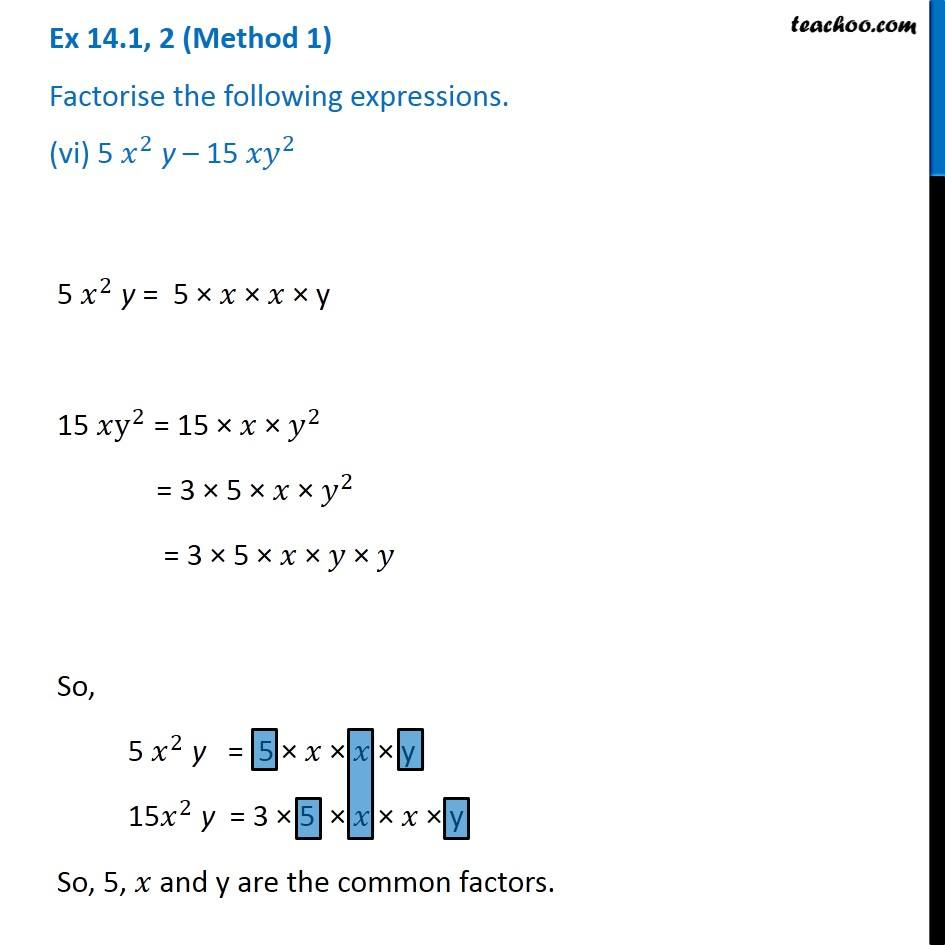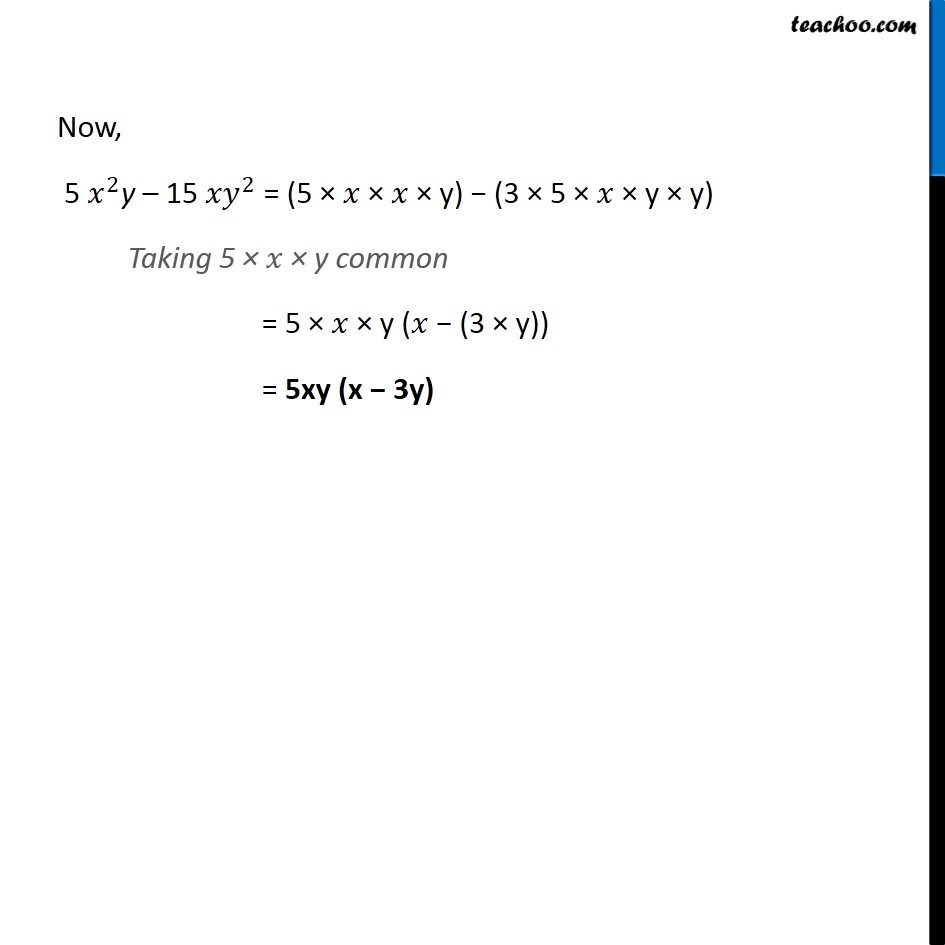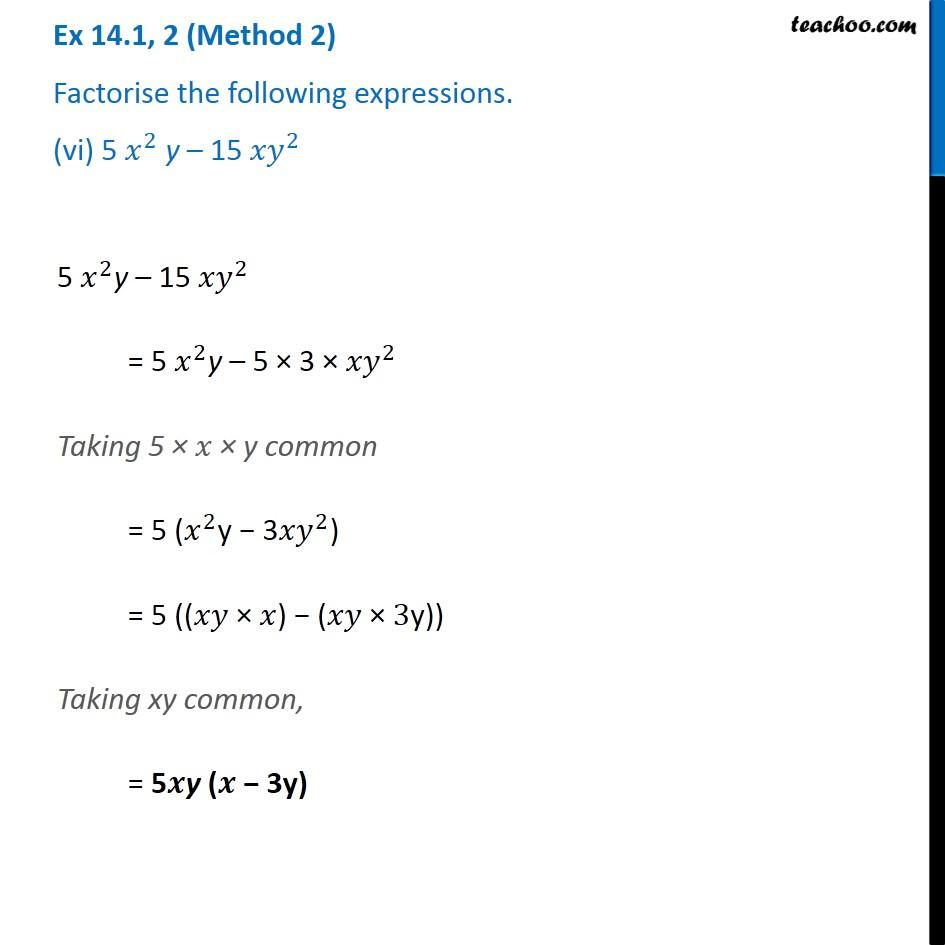Factorisation using common factors

Chapter 12 Class 8 Factorisation
Concept wiseGet live Maths 1-on-1 Classs - Class 6 to 12

### Transcript

Ex 12.1, 2 (Method 1) Factorise the following expressions. (vi) 5 𝑥^2 y – 15 〖𝑥𝑦〗^2 5 𝑥^2 y = 5 × 𝑥 × 𝑥 × y 15 𝑥y^2 = 15 × 𝑥 × 𝑦^2 = 3 × 5 × 𝑥 × 𝑦^2 = 3 × 5 × 𝑥 × 𝑦 × 𝑦 So, 5 𝑥^2 y = 5 × 𝑥 × 𝑥 × y 15𝑥^2 y = 3 × 5 × 𝑥 × 𝑥 × y So, 5, 𝑥 and y are the common factors. Now, 5 𝑥^2y – 15 〖𝑥𝑦〗^2 = (5 × 𝑥 × 𝑥 × y) − (3 × 5 × 𝑥 × y × y) Taking 5 × 𝑥 × y common = 5 × 𝑥 × y (𝑥 − (3 × y)) = 5xy (x − 3y) Ex 12.1, 2 (Method 2) Factorise the following expressions. (vi) 5 𝑥^2 y – 15 〖𝑥𝑦〗^2 5 𝑥^2y – 15 〖𝑥𝑦〗^2 = 5 𝑥^2y – 5 × 3 × 〖𝑥𝑦〗^2 Taking 5 × 𝑥 × y common = 5 (𝑥^2y − 3〖𝑥𝑦〗^2) = 5 ((𝑥𝑦 × 𝑥) − (𝑥𝑦 × 3y)) Taking xy common, = 5𝒙y (𝒙 − 3y)